# N-gon + triangle - math problems

Examples of a regular polygon (polygon). Polygon is a closed part of the plane bounded by a broken line. Points that define a polygon are the polygon vertices. The number of vertices, sides, and interior angles in a polygon is the same, and this number specifies the name of the polygon: triangle, quadrangle, pentagon, etc.

1. Five-gon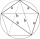Calculate the side a, the circumference and the area of the regular 5-angle if Rop = 6cm.
2. AnnulusTwo concentric circles with radii 1 and 9 surround the annular circle. This ring is inscribed with n circles that do not overlap. Determine the highest possible value of n.
3. Top of the towerThe top of the tower has the shape of a regular hexagonal pyramid. The base edge has a length of 1.2 m, the pyramid height is 1.6 m. How many square meters of sheet metal is needed to cover the top of the tower if 15% extra sheet metal is needed for joint
4. Hexagonal pyramidCalculate the surface area of a regular hexagonal pyramid with a base inscribed in a circle with a radius of 8 cm and a height of 20 cm.In a regular quadrilateral pyramid, the side edge is e = 7 dm and the diagonal of the base is 50 cm. Calculate the pyramid shell area.
6. Triangular pyramidA regular tetrahedron is a triangular pyramid whose base and walls are identical equilateral triangles. Calculate the height of this body if the edge length is a = 8 cm
7. Wall heightCalculate the height of a regular hexagonal pyramid with a base edge of 5 cm and a wall height w = 20 cm.
8. Hexa pyramidThe base of the regular pyramid is a hexagon, which can be described by a circle with a radius of 1 m. Find the volume of the pyramid 2.5 m high.
9. Octagonal pyramidFind the volume of a regular octagonal pyramid with height v = 100 and the angle of the side edge with the plane of the base is α = 60°.
10. Hexagonal pyramidPlease calculate the height of a regular hexagonal pyramid with a base edge of 5cm and a wall height of w = 20cm. Please sketch a picture.
11. Hexagon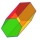Calculate the surface of a regular hexagonal prism whose base edge a = 12cm and side edge b = 3 dm.
12. Octagonal tank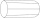The tank has the shape of a regular octagonal prism without an upper base. The base edge has a = 3m, the side edge b = 6m. How much metal sheet is needed to build the tank? Do not think about losses or sheet thickness.
13. MO Z8–I–6 2018In the KLMN trapeze, KL has a 40 cm base and an MN of 16 cm. Point P lies on the KL line so that the NP segment divides the trapezoid into two parts with the same area. Find the length of the KP line.
14. Diagonals of pentagonCalculate the diagonal length of the regular pentagon: a) inscribed in a circle of radius 12dm; b) a circumscribed circle with a radius of 12dm.
15. NonagonCalculate the area and perimeter of a regular nonagon if its radius of inscribed circle is r = 10cm
16. DecagonCalculate the area and circumference of the regular decagon when its radius of a circle circumscribing is R = 1m
17. The perimeter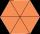The perimeter of equilateral △PQR is 12. The perimeter of regular hexagon STUVWX is also 12. What is the ratio of the area of △PQR to the area of STUVWX?
18. In a 2In a thirteen sided polygon, the sum of five angles is 1274°, four of the eight angles remaining are equal and the other four are 18° less than each of the equal angles. Find the angles. .
19. Six-sided polygon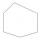In a six-sided polygon. The first two angles are equal, the third angle is twice (the equal angles), two other angles are trice the equal angle, while the last angle is a right angle. Find the value of each angle.
20. Hexagon ABCDEFIn the regular hexagon ABCDEF, the diagonal AE has a length 8cm. Calculate the circumference and the hexagon area.

Do you have an interesting mathematical word problem that you can't solve it? Enter it, and we can try to solve it.

We will send a solution to your e-mail address. Solved examples are also published here. Please enter the e-mail correctly and check whether you don't have a full mailbox.

Please do not submit problems from current active competitions such as Mathematical Olympiad, correspondence seminars etc...